## Chapter 5. Data Representation and Storage

This chapter shows how different data types are represented in storage and describes how the compiler uses storage.

Numbers shown on the formats are bit positions, which represent powers of 2 in binary notation. Code that depends on internal representation is not portable and might not conform with the Fortran standard.Note:: Storage words are represented here with bits counted from the right, making bit 0 the low-order bit and bit 31 or 63 the high-order bit.

This chapter describes the machine representation of data. The last sections in this chapter describe storage issues, including overindexing.

## 5.1. Data Representation for IRIX systems

On IRIX systems KIND=4 values are stored in 32 bits and can be packed two per word.

### 5.1.1. Integer Type

The following sections describe integer data representation of KIND=1 , 2, 4, and 8.

#### 5.1.1.1. KIND=1

Range: -27 < I < 2 7 or approximately -102 < I < 10 2

Figure 5-1. INTEGER(KIND=1)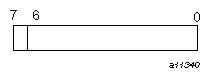To declare 8-bit integers, specify one of the following:

• KIND=1.

• KIND=KIND(kind_expr) , where kind_expr is a scalar initialization expression with a kind type parameter that evaluates to 1.

#### 5.1.1.2. KIND=2

Range: -215 < I < 2 15 or approximately -104 < I < 10 4

Figure 5-2. INTEGER(KIND=2)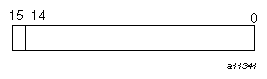To declare 16-bit integers, specify one of the following:

• KIND=2.

• KIND=KIND(kind_expr), where kind_expr is a scalar initialization expression with a kind type parameter that evaluates to 2.

#### 5.1.1.3. KIND=4

Range: -231 < I < 2 31 or approximately -109 < I < 10 9

Figure 5-3. INTEGER(KIND=4)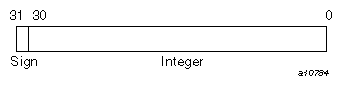To declare 32-bit integers, specify one of the following:

• KIND=4.

• KIND=KIND(kind_expr), where kind_expr is a scalar initialization expression with a kind type parameter that evaluates to 4.

#### 5.1.1.4. KIND=8

Range: -263 < I < 2 63 or approximately -1018 < I < 10 18

Figure 5-4. INTEGER(KIND=8)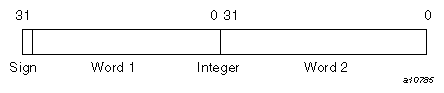To declare 64-bit integers, specify one of the following:

• KIND=8.

• KIND=KIND(kind_expr), where kind_expr is a scalar initialization expression with a kind type parameter that evaluates to 8.

### 5.1.2. Real Type

The following sections describe real data representation of KIND= 4, 8, and 16. Real (floating-point) numbers are represented in a packed representation of a sign, an exponent (power of 2), and a binary mantissa.

#### 5.1.2.1. KIND=4

Range: -2-125 .LE. I < 2 128 or approximately -10-38 .LE. I < 10 38

Figure 5-5. REAL(KIND=4)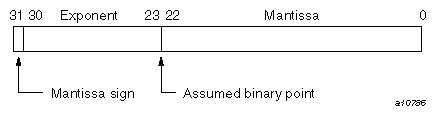To declare 32-bit reals, specify one of the following:

• KIND=4.

• KIND=KIND(kind_expr ), where kind_expr is a scalar initialization expression with a kind type parameter that evaluates to 4.

Notes on real data type representation:

The exponent is a power of 2, represented by a number that is 177 8 higher than the actual value; this is called a bias . The effect of the bias is that the second bit in the word serves as the exponent's sign bit. This bit's usage is the inverse of the mantissa's sign bit, as follows:

 Bit Applies to 1 value indicates 31 Mantissa Negative 30 Exponent Positive ( > 0 )

The exponent is represented by the second through ninth digits in a binary printout; these digits have the range 011111112 through 111111102 for a positive exponent, and 00000000 2 through 011111102 for a negative exponent.

When the bias is accounted for, the range of all exponents is as follows:

• 2-177 to 2 177 (octal)

or

• 2-127 to 2 127 (decimal)

The mantissa is a 24-bit fraction with an assumed leading 1; that is, the leading 1 is not stored. The only exception is for the value 0, which has an assumed leading 0. The sign of the mantissa is separated from the rest of the mantissa as shown in the preceding diagram. The mantissa is not complemented for negative values. That is, the mantissa for -10.0 is the same as for +10.0.

In terms of decimal values, the 32-bit floating-point format allows representation of numbers to about 7 significant decimal digits in the following approximate decimal range:

1.18 × 10-38 < R < 3.4 × 10 38

A zero value is not biased and is represented as a word of all zeros.

The following are some sample numbers as represented within memory:

Decimal

Octal

10.0

010110000000

41200000

-10.0

030110000000

C1200000

0.1

007563146315

3DCCCCCD

-0.1

027563146315

BDCCCCCD

Figure 5-6. Binary version of 10.0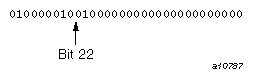The leftmost bit, with a 0 value, indicates a positive mantissa; that is, the real value is positive. The next 8 bits (10000010, or decimal 130) are the exponent. Subtracting the bias of 127 yields an exponent of 3, meaning that the binary fraction in the mantissa is multiplied by 23 . To express it another way, the binary point is moved 3 bits to the right from the mantissa's highest bit. Interpreted this way, the first 4 bits of the mantissa, 010, indicate the real decimal value 10.0 (remember that there is an assumed 1 to the left of the mantissa in the IEEE floating-point format with a binary point to its immediate right). You can display other values by printing them with formats O11, Z8, or B32.

#### 5.1.2.2. KIND=8

Double precision, REAL(KIND=8), values are represented in 2 words.

Range: -2-1021 .LE. I < 2 1024 or approximately -10-308 .LE. I < 10 308

Figure 5-7. REAL(KIND=8)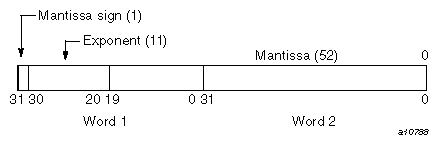To declare 64-bit reals, specify one of the following:

• KIND=8.

• KIND=KIND(kind_expr), where kind_expr is a scalar initialization expression with a kind type parameter that evaluates to 8.

#### 5.1.2.3. KIND=16

Quad precision, REAL(KIND=16), values are represented in 4 words. For more information on quad precision representation, see math(3m) (note that “long double” is a synonym for quad precision).

Range: -2-967 .LE. I < 2 1023 or approximately -10-292 .LE. I < 10 308

Figure 5-8. REAL(KIND=16)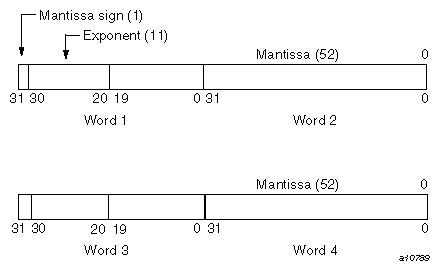To declare 128-bit reals, specify one of the following:

• KIND=16.

• KIND=KIND(kind_expr ), where kind_expr is a scalar initialization expression with a kind type parameter that evaluates to 16.

### 5.1.3. Complex Type

The following sections describe complex data representation of KIND=4 , 8, and 16. A complex value has two parts. The first part represents the real part, and the second represents the imaginary part. Each part has the same range as a real value.

#### 5.1.3.1. KIND=4

A single-precision, KIND=4, complex value is represented by 2 parts. The first part represents the real part, and the second represents the imaginary part. Each part has the same range as a real value.

Range: -2-125 .LE. I < 2 128 or approximately -1038 .LE. I < 10 38

Figure 5-9. COMPLEX(KIND=4) (real portion)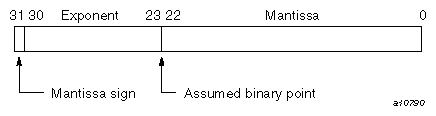Figure 5-10. COMPLEX(KIND=4) (imaginary portion)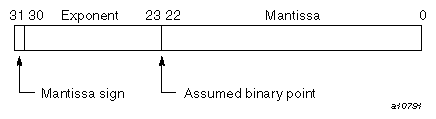To declare an entity to be of single-precision, complex type, specify one of the following:

• KIND=4.

• KIND=KIND(kind_expr), where kind_expr is a scalar initialization expression with a kind type parameter that evaluates to 4.

#### 5.1.3.2. KIND=8

A double-precision, KIND=8, complex value is represented by 4 words. The first 2 words represent the real part, and the second 2 words represent the imaginary part. Each word has the same range as a real value.

Range: -2-1021 .LE. I < 2 1024 or approximately -10308 .LE. I < 10 308

Figure 5-11. COMPLEX(KIND=8) (real portion)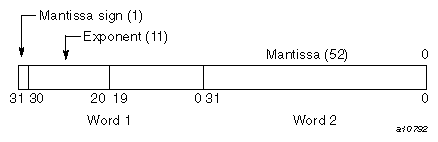Figure 5-12. COMPLEX(KIND=8) (imaginary portion)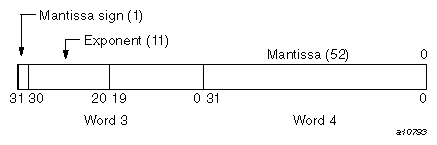To declare an entity to be of double-precision, complex type, specify one of the following:

• KIND=8.

• KIND=KIND(kind_expr), where kind_expr is a scalar initialization expression with a kind type parameter that evaluates to 8.

#### 5.1.3.3. KIND=16

A quad precision, KIND=16, complex value is represented by 8 words. The first 4 words represent the real part, and the second 4 words represent the imaginary part. Each word has the same range as a real value.

Range: -2-967 .LE. I < 2 1023 or approximately -10-292 .LE. I < 10 308

Figure 5-13. COMPLEX(KIND=16) (real portion)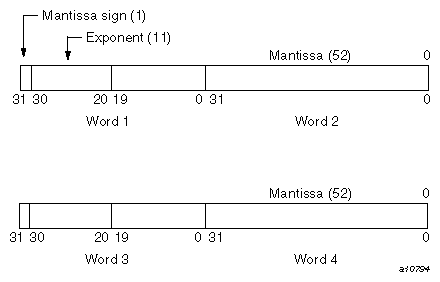Figure 5-14. COMPLEX(KIND=16) (imaginary portion)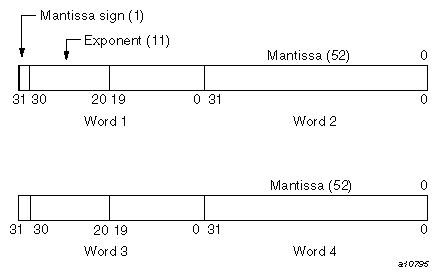To declare an entity to be of quad precision, complex type, specify one of the following:

• KIND=16.

• KIND=KIND(kind_expr ), where kind_expr is a scalar initialization expression with a kind type parameter that evaluates to 16.

### 5.1.4. Character Type

Characters are represented by 8-bit ASCII codes. The codes are stored in 1 byte.

Figure 5-15. Character type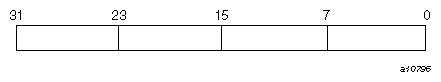The compiler does not support a nondefault character type. The only kind value supported is 1.

### 5.1.5. Logical Type

Logical entities specified as KIND=1, KIND=2, and KIND=4 occupy 32 bits . Logical entities specified as KIND=8 occupy 64 bits on IRIX systems. Its value is true if the numeric value in the word is one (1). Its value is false if the numeric value in the word is zero (0).Note:: SGI does not guarantee a particular internal representation of logical values on any machine or system; the compiler is designed on the assumption that logical values will be used only as described in the Fortran standard. Therefore, it is not good programming practice to use logical values as numbers or vice versa.

To declare an entity to be of logical type, you can specify one of the following:

• KIND=1, KIND=2, KIND=4, or KIND=8.

• KIND=KIND(kind_expr), where kind_expr is a scalar initialization expression with a kind type parameter that evaluates to 1, 2, 4, or 8.

## 5.2. Storage Issues

This section describes how the compiler uses storage, including how the compiler accommodates programs that use overindexing.Note:: The information in this section assumes that you are using the default data representations.

The following options to the f90(1) command affect storage and data representation:

• -d16 changes default double precision and double complex to 128 bits

• -i4 changes default integer and logical to 32 bits

• -i8 changes default integer and logical to 64 bits

• -n32 and -64 change pointer sizes and the maximum amount of addressable memory

• -r4 makes default real and complex be 32 bits/64 bits (default)

• -r8 changes default real and complex to 64 bits/128 bits

### 5.2.1. Storage Units and Sequences

A numeric storage unit can be one of the following:

• A word on systems of 32 bits.

A character storage unit is an 8-bit byte.

A storage sequence is a contiguous group of storage units with a consecutive series of addresses. Each array and each common block is stored in a storage sequence. The size of a storage sequence is the number of storage units it contains. Two storage sequences are associated if they share at least one storage unit.

Complex values occupy twice the storage of real values. The real portion of the complex value occupies the first half of the total storage; the imaginary portion of the complex value occupies the second half of the total storage, as follows:

• A double-precision value uses a storage sequence of 8 or 16 bytes. Depending on the KIND= specification, a complex value uses 8, 16, or 32 bytes. The first half of the bytes used contains the most significant bits of a double-precision value or the real part of a complex value. The last half of the bytes used contains the least significant bits of a double-precision value or the imaginary part of a complex value.

• A double-complex value occupies 16 bytes of storage; the first 8 bytes contain the real part of the complex value, and the second 8 bytes contain the imaginary part.

A quad precision complex value occupies 32 bytes of storage; the first 16 bytes contain the real part of the complex value, and the second 16 bytes contain the imaginary part.

A character value is represented as an 8-bit ASCII code, packed 4 characters per byte. The storage size depends on the length specification of the value.Note: The Fortran standard does not specify the relationship between storage units and computer words, and it does not specify any relation between default numeric and character storage units.

### 5.2.2. Static and Stack Storage

With static storage, any variable that is allocated memory occupies the same address throughout program execution. Allocation is determined before program execution.

Code using static storage can be used with Autotasking, multitasking, and macrotasking if variables in static storage conform to the following guidelines:

• Loops are Autotasked regardless of the presence of variables in static or stack storage. Scoping is controlled by the presence of PRIVATE or SHARED parameters on the DOALL Autotasking directive. If a subroutine that contains static data is called from within an autotasked loop, static data is treated as shared data, which means that the static data must be protected by GUARD and ENDGUARD Autotasking directives.

• Variables in static storage can be read when loops are multitasked and macrotasked. If a loop modifies variables in static storage, you must use guards (GUARD and ENDGUARD Autotasking directives) or locks (LOCKON() and LOCKOFF() calls) to protect the variables.

Stack storage is the default for all subprograms, but static storage is the default for items that require 256 bits of storage in a main program. The stack is an area of memory where storage for variables is allocated when a subprogram or procedure begins execution. These variables are released when execution completes. The stack expands and contracts as procedures are entered and exited. Autotasking and recursion require a stack.

When stack storage is used, the value of a variable is not saved between invocations of a subprogram unless it is specified in a SAVE or DATA statement. When f90 -static is specified, all user variables are treated as if they appeared in a SAVE statement. When -e v or -static is in effect, compiler-generated temporary variables and the calling sequence are still allocated to the stack.

A heap is memory that, like a stack, is dynamically allocated; it is used internally.

The compiler allocates variables to storage according to the following criteria:

• Variables in common blocks are always allocated in the order in which they appear in COMMON statements.

• Data in modules are statically allocated.

• User variables that are defined or referenced in a program unit, and that also appear in SAVE or DATA statements, are allocated to static storage, but not necessarily in the order shown in your source program.

• Other referenced user variables are assigned to the stack. If -static is specified on the f90(1) command line, referenced variables are allocated to static storage. This allocation does not necessarily depend on the order in which the variables appear in your source program.

• Compiler-generated variables are assigned to a register or to memory (to the stack or heap), depending on how the variable is used. Compiler-generated variables include DO-loop trip counts, dummy argument addresses, temporaries used in expression evaluation, argument lists, and variables storing adjustable dimension bounds at entries.

• Automatic objects may be allocated to either the stack or to the heap, depending on how much stack space is available when the objects are allocated.

• Heap or stack allocation can be used for TASK COMMON variables and some compiler-generated temporary data such as automatic arrays and array temporaries.Note:: Unreferenced user variables not appearing in COMMON statements are not allocated.